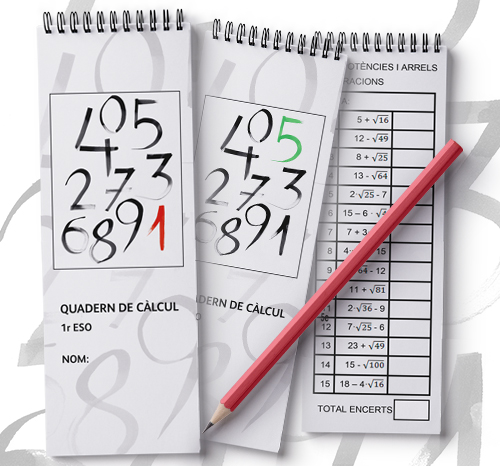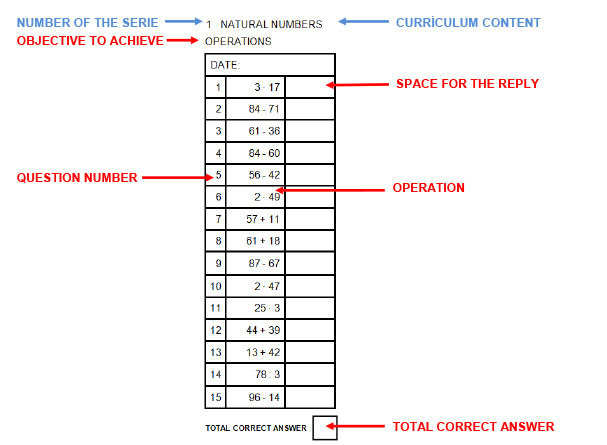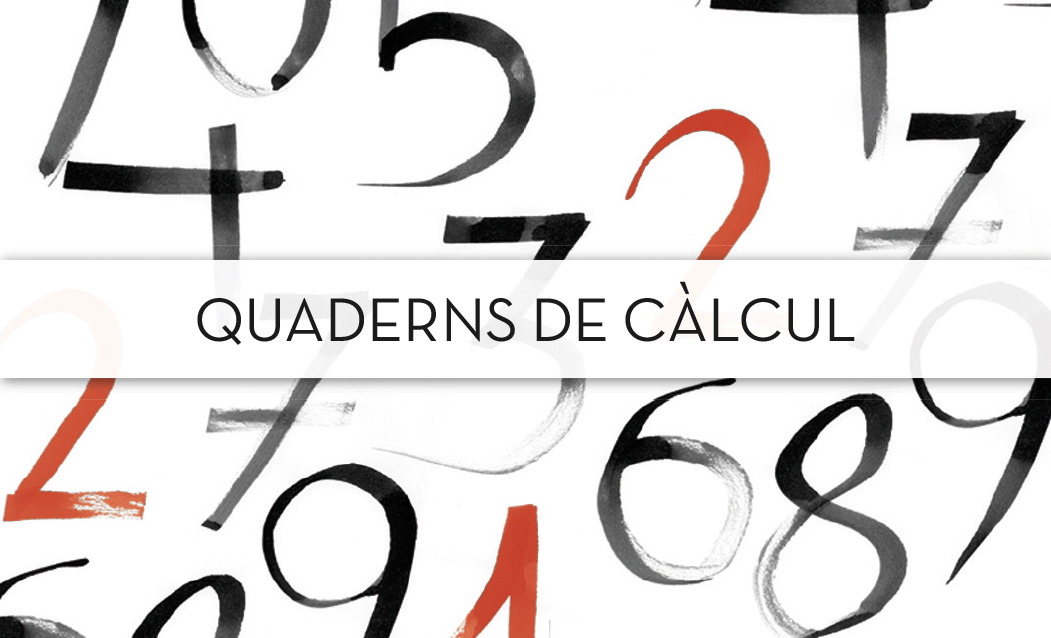A PERFECT TOOL FOR SHARPENING MENTAL CALCULATION

DISCOVER THEM!

## CALCULATION workbooks

### These calculus workbooks arise from the need to develop specific materials which could help the students improve certain mathematical skills such as mental calculation and algorithmic calculation, and that, at the same time are interrelated to the contents of the curriculum.

The main aims that are intended to achieve are:

• Boosting student’s attention and concentration.
• Using strategies to memorize and retrieve information.
• Master the contents of the subject such as:
- Progressively knowing and using the different types of numbers.
- Using the different properties when performing operations…## DESCRIPTION OF THE MATERIALS: EXPLANATION OF THE CALCULATION NOTEBOOK

### Each calculation notebook consists of a collection of calculation worksheets with operations related to the contents that will be worked in class every day.

The worksheets are classified into four blocks: Arithmetic and Calculation, Algebra, Geometry and Functions, being the part of Arithmetic and Calculation the one which has a major presence.

### SECUNDARIA

In each series we will find:• The content of the curriculum (Natural numbers, Powers, Fractions ...)..
• Which objective to practise (Operations, Properties, Calculation of percentages, Equations...).
• Space for the date.
• The number of each question (15 in total).
• The operation to perform.
• The total number of correct answers.

At the end of the notebook we will find a grid for each term, to build the frequency polygon of the number of the right answers of each day. In this way the students will be aware of their progress.### These materials have been prepared to be worked everyday at the beginning of the lesson.

It is designed so that the students solve each series in a certain time (one minute; one minute and a half; two minutes ... depending on the level of the students in each group). But we must insist that the success of this activity consists of getting as many correct results as possible, and not the speed with which it is carried out.

### The practice must be constant. It is necessary to repeat the procedures many times to achieve agility.

As the results improve, students gain confidence and self-assurance.
After making the correction, we can give the option to review the results to see where they were wrong.

## WHAT DO WE EXPECT FROM STUDENTS?

### We want students build their own personal calculation strategies that allow them to solve more complex operations.

These materials are aimed at all kinds of students, either those who are very skilled in calculating or those who are not. With these last ones it is necessary to insist on the fact that daily practice will help them get good results. They need to be constant every day and thus they will find an improvement in the mathematical performance, which will increase their self-esteem and the excitement to go on.

## HOW DO WE WORK THE CALENDAR CLASS?

• ##### 1.

Every day at the beginning of the class, we distribute the workbooks to the students and we give them instructions on the series that they have to solve.

#### 2.

Students prepare their pens, open the notebook on the corresponding page and write down the date.

• ##### 3.

EWe indicate the content to be worked on and what the activity consists of. We start the stopwatch.

#### 4.

When stopwatch finishes, students stop writing and prepare the red pen to make the correction.

• ## 5

We use the answer key to tell the students the right answers while students correct their own exercises

#### 6.

After finishing, each student counts the correct answers and writes the result in the corresponding box. Then they mark it on the grid of the end of the notebook.

• ## 7

Finally, we keep a register of the results that each student obtains and we collect the workbooks

## IDEAS AND SUGGESTIONS

• We can use the materials to adapt them to our own curricular needs, changing the timing or the order of the series.
• Sometimes it is interesting to make two worksheets one after the other to check if the students have achieved the content that we are working on.
• The day of the written test it is not necessary to make calculations.
• To facilitate the availability of this material, it can be kept in class.

## ASSESSMENT OF THE STUDENTS OF THIS MATERIAL

• It improves the speed in solving operations.
• It helps me to revise and reinforce what we are working in class and understand it the better
• Making calculations at the beginning of the class helps me focus during the rest of the lesson
• Having practiced calculus makes me feel more confident
• When homework exercises are related to calculation, i find them easier to do
• I love calculation. It helps me understand problems more easily and I find it even fun
• Calculation has been helpful for me to focus and understand things
• It has helped me to improve the results of the first term
• With calculation we revise topics from former lessons
• I have finally understood things which I found difficult to understand, through daily practice.

## contacta conmigo

### Maria Bas Tortosa

Bachelor of Mathematics and Secondary School teacher

600 45 80 83

### email

mbastor@gmail.com